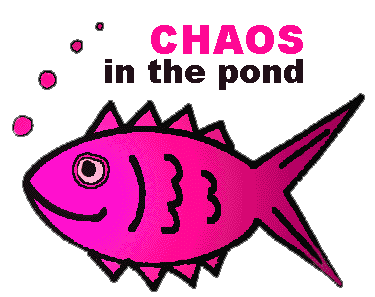Source: James Gleick's Chaos, Making a New ScienceIMAGINE A pond with goldfish swimming around in it. Each year, you go out to the pond and count the number of fish. Some years the number is similar to number you got last year; sometimes it's completely different. Assuming you're the kind of fish-keeper with a mathematics fetish, how do you go around modelling your fish population?

One model is to assume that your fish stock increases each year by some growth rate:

``` 	y(next year) = rate * y(this year)
```
...where "y" is the number of fish and "rate" is the growth rate (say, 2.4).

Of course the problem is that the pond is only so big, and there only so much food around. There has to be something in the model to limit the population growth. Otherwise you'll be up to your neck in goldfish in no time.

Enough of the lecture, jump me straight to...

• The logisitc equation graphing applet.
• Bifurcation graph applet.